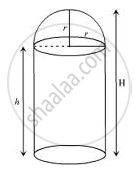Advertisement Remove all ads

# A Building is in the Form of a Cylinder Surmounted by a Hemi-spherical Vaulted Dome and Contains 41 19 21 M 3 of Air - Mathematics

Answer in Brief

A building is in the form of a cylinder surmounted by a hemi-spherical vaulted dome and contains  $41\frac{19}{21} m^3$ of air. If the internal diameter of dome is equal to its total height  above the floor , find the height of the building ?

Advertisement Remove all ads

#### Solutionlet the total height of the building be H m.

let the radius of the base be r m. Therefore the radius of the hemispherical dome is r m.

Now given that internal diameter = total height

$\Rightarrow 2r = H$

Total height of the building = height of the cylinder +radius of the dome
⇒ H = h + r
⇒ 2r = h + r
⇒ r = h

Volume of the air inside the building = volume of the cylinder+ volume of the hemisphere

$\Rightarrow 41\frac{19}{21} = \pi r^2 h + \frac{2}{3} \pi r^3$

$\Rightarrow \frac{880}{21} = \pi h^2 h + \frac{2}{3} \pi h^3$

$\Rightarrow \frac{880}{21} = \pi h^3 \left( 1 + \frac{2}{3} \right)$

$\Rightarrow \frac{880}{21} = \pi h^3 \left( \frac{5}{3} \right)$

$\Rightarrow h = 2 m$

Hence, height of the building H = 2 × 2 = 4m

Is there an error in this question or solution?
Advertisement Remove all ads

#### APPEARS IN

RD Sharma Class 10 Maths
Chapter 14 Surface Areas and Volumes
Exercise 14.2 | Q 34 | Page 62
Advertisement Remove all ads
Advertisement Remove all ads
Share
Notifications

View all notifications

Forgot password?